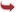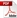International Journal of Scientific and Research Publications

#### IJSRP, Volume 10, Issue 6, June 2020 Edition [ISSN 2250-3153]Application of Excel Spreadsheet for comparative analysis of linearized expressions of kinetics modelling for the adsorption of methylene blue on rice husk
Yu-Ting Huang, Ming-Cheng Shih
Abstract: In order to explore the feasibility of six different linear conversion methods applied to nonlinear pseudo-second-order kinetic equations, six linear expressions are derived from these different conversion methods. These linear expressions are applied to the experimental data and used the mathematical and statistical data obtained from the calculation of these 6 linear expressions to analyze and determine which linear expression calculated the data closest to the actual experimental data than the other linear expressions. Most computers are equipped with EXCEL, so it is the most suitable tool for researchers to apply the EXCEL on these linear equations for various calculations and statistical analysis. Therefore, the Data Analysis toolpak, an analysis tool incorporated into Microsoft Excel for Windows, was used to analyze and find the best linear type fitted to experimental data from 6 different types of linear expression of pseudo-second-order kinetic equation.
[VIEW FULL PAPER][DOWNLOAD]

Reference this Research Paper (copy & paste below code):

Yu-Ting Huang, Ming-Cheng Shih (2020); Application of Excel Spreadsheet for comparative analysis of linearized expressions of kinetics modelling for the adsorption of methylene blue on rice husk; International Journal of Scientific and Research Publications (IJSRP) 10(06) (ISSN: 2250-3153), DOI: http://dx.doi.org/10.29322/IJSRP.10.06.2020.p10265